# How would you get the position of a normal

I made a placement system its already almost done but ran into a mathematical problem
how would you get a part position to rotate off a normal I’m using normal because I have rotation mechanics in my placement system my guess is a trigonometry function (sine,cosine,tangent) but don’t know which one I should use I never really use trigonometry functions very often
any help will be appreciate heres some more depth in my question

you see when they are both 0,0,0 orientation the placement system works good!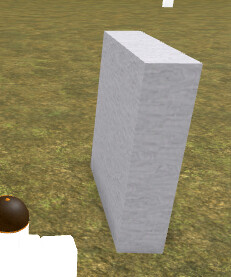but once you rotate one of them
theres a weird position offset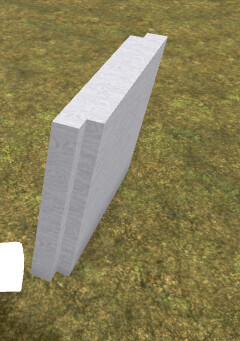Again any help will be appreciated

2 Likes

You might be looking for Up Vector, Right Vector, and Look Vector.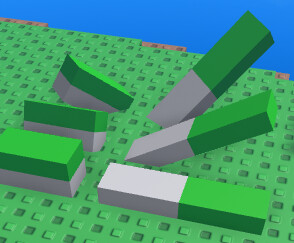The gray parts on the left side were positioned/rotated manually. The green parts are clone made with this offset applied to them:

``````part.CFrame = (part.CFrame + part.CFrame.UpVector *1)
``````

‘1’ is the y value of the size of the part

Similarly the gray parts on the right were positioned/rotated manually. The green parts are a clone made with this offset applied to them:

``````part.CFrame = (part.CFrame - part.CFrame.RightVector * 4) -- The '-' made it technically (LeftVector)
``````

‘4’ is the X value of the size of the part

Documentation is found here:
https://developer.roblox.com/en-us/api-reference/datatype/CFrame

To get your normal position, you’ll just need to take the appropriate Vector (Look, Up, Right) and * it by 1/2 the appropriate X, Y, or Z of the size.

3 Likes

Correct me if I’m wrong but that is what I did

``````local ActivedObjectCFrame = ActivedObject.Parent:GetPrimaryPartCFrame()
clientmodel:SetPrimaryPartCFrame(CalculatedCFrame)
--The ActivedObject is the part the mouse is on
--The MouseResults is a raycast from camera to mouse
``````

1 Like

It took me a while, but I was able to reproduce the same weird offset results.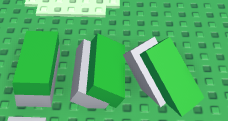``````part.CFrame = CFrame.new(part.CFrame * part.CFrame.UpVector)
``````

VS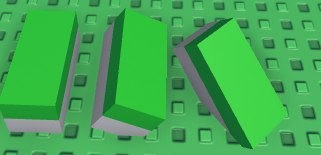``````part.CFrame = part.CFrame + part.CFrame.UpVector
``````

I’m not going to lie, ray casts and complicated equations are foreign to me I can only tell you what worked for me, and what didn’t. There are people out there who would do much better then me explaining what’s going on here.

3 Likes

Thanks for the help anyways if anyone can find the reason for this I will appreciate it I have been trying to make this placement system work for a while now barely got it to snap on other parts a couple of hours ago after 2 weeks of researching

If I wasn’t clear (sorry) I’m fairly certain you’re equation would look something like this.

``````local CalculatedCFrame = CFrame.new(ActivedObjectCFrame + (MouseResults.Normal*ActivedObject.Parent:GetExtentsSize())))* CFrame.Angles(math.rad(ComputedRotation.X),math.rad(ComputedRotation.Y),math.rad(ComputedRotation.Z))
``````
1 Like
``````local CalculatedCFrame = CFrame.new(ActivedObjectCFrame.Position+(MouseResults.Normal*ActivedObject.Parent:GetExtentsSize()))* CFrame.Angles(math.rad(ComputedRotation.X),math.rad(ComputedRotation.Y),math.rad(ComputedRotation.Z))
``````

Assuming you grouped the model, you can probably derive the position of the center of the model, and depending on how far a part is from that center in 3d space, use some trigo to calculate how much you would need to offset the position to match with the rotation.

apologies for my atrocious drawing, just hope it clarifies some things.

3 Likes

Ok, I’ve discovered a second issue with the code.

Before I get into it I want to explain what a Directional Vector is.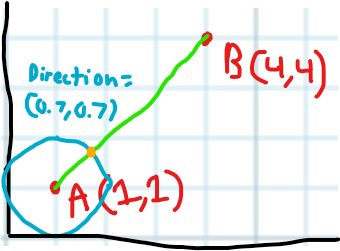Here you can see that the directional vector from point A to point B is (0.7, 0.7)
It’s actually (0.7071…, 0.7071…)
It’s the distance traveled in both x and y, to reach exactly 1 stud away from point A to point B.

Ok understanding that to move point A in the direction of B exactly 3 studs away we would take A (1,1) and add (Directional Vector(0.7, 0.7) * the # of studs(3))

``````local A = Vector2.new(1,1)
local DirectionalVector = Vector2.new(0.7071...,0.7071...)
local A1 =  A + DirectionalVector * 3
``````

The Directional Vector is what you get by MouseResults.Normal, or CFrame.UpVector.

So understanding that we can get into the problem. We’re using :GetExtentsSize() in order to find the #of studs, Just so we’re clear Multiplying Vectors together looks like this:

``````Vector3.new(2, 4, 3) * Vector3.new(4, 1, 2)
-- Gives:
Vector3.new(8, 4, 6)
``````

Lets try this with what we’ve learned so far.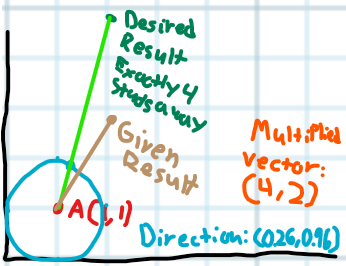Lets assume the parts size is 4,2, and the directional vector we’ve already calculated is (0.26, 0.96) and our first point A is (1,1). If we multiply the directional vector by (4, 2) we get the brown point (because 0.96 * 2) is just under 2 studs. when just multiplying it by (4, 4) (or just 4) gives us the green point.
so if we take this and plug it into the equation you’re using we get:

``````local NewPoint = ACFrame + DirectionalVector * Vector2.new(4, 2)
``````

Ok, so the problem here is that the Directional Vector is how far to move the point in each direction to make it 1 stud away, when moving it 4 studs away we would want to just multiply it by 4, however, we’re multiplying it by (4,2) its as if the x value was aiming for 4 studs away, and the y value was only aiming for 2 studs away.

So hopefully I’ve clearly communicated that the problem lies in multiplying the Direction by the Vector3, when it should only be multiplied by the distance away it needs to be, now the question we need to figure out is how to find which distance to use. It’s quite obvious when using UpVector, LookVector, or RightVector, because UpVector is connected to the Y value of scale. However in this case we don’t have that abililty.

So we need to figure out which face we’re trying to attach to, (Front, Top, Right, etc…) From what I’ve found the correct way it to compare our directionalVector against all of the faces directional vector. If they’re the same (or extremely close due to floating point) we’ll have found the correct face.

This post explains it well:

After we’ve found which face it’s gone through we just need to connect each face to the corresponding X, Y, or Z value of the Size.

Front and Back correlate to the X value, Top and Bottom correlate to the Y value, and Left and Right correlate to the Z value.

Hopefully this is enough information to be able to build up the fixed function on your own, if you need help feel free to DM me, and I’ll make sure you get the correct result.

Thank you for posting this problem, I had a lot of fun and learned a lot from finding the solution. I hope it helped!Edit: Oh btw in your video it looks as if you’re drawing the ray from the character to the mouse, when it’d look more accurate if you drew it from the cameras position to the mouse.

5 Likes